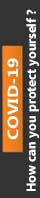We're Open

0% Plagiarism Guaranteed & Custom Written

# Q1 Use the Newton Raphson Method to determine √7 3 correct to 7 decimal places by considering the function 𝑓𝑓(𝑥𝑥) = 𝑥𝑥3 − 7.

MATH51000

Assignment 1

You are required to produce a Maple or Mupad worksheet which answers the accompanying questions. As part of your answers you should include text describing, in your own words, what you have done. You should export your worksheet as a pdf document. You should submit the pdf and Maple/Mupad document via the assignment link on Blackboard.

Q1 Use the Newton Raphson Method to determine √7 3 correct to 7 decimal places by considering the function 𝑓𝑓(𝑥𝑥) = 𝑥𝑥3 − 7.

Q2 The Colebrook equation is used for turbulent flows through rough pipes. The Colebrook equation is 1 �𝑓𝑓 = −2 log10 � 𝜀𝜀 14.8 Rh + 2.51 Re √f �,

where f is the Darcy friction factor; ε is the roughness height; Rh is the hydraulic radius and Re is the Reynolds number. Use the following values: Re = 4900, ε = 0.0045 and Rh = 0.15.

(i) Form an appropriate function g (f ) to be used in the Newton Raphson method and plot it.

(ii) Use your graph to determine an initial approximation to the friction factor

(iii) Use the Newton Raphson method to determine the friction factor correct to 7 significant figures

Q3 Calculate the determinant to determine which of the following equations have unique solutions. (i) 5 𝑥𝑥 + 3 𝑦𝑦 = 2 2 𝑦𝑦 − 4 𝑥𝑥 = 3 (ii) 2 𝑥𝑥 − 𝑦𝑦 − 𝑧𝑧 = 2 3 𝑥𝑥 − 2 𝑦𝑦 + 2 𝑧𝑧 = −2 − 𝑥𝑥 + 𝑦𝑦 − 3 𝑧𝑧 = 3 (iii) 4 𝑥𝑥 − 2𝑦𝑦 + 3 𝑧𝑧 = 3 2 𝑥𝑥 − 𝑦𝑦 + 𝑧𝑧 = −2 2 𝑥𝑥 − 3 𝑦𝑦 + 2 𝑧𝑧 = −1 Use the inverse matrix to solve the equations where possible

Q4 Find the 2 values of λ so that the following equations do not have a unique solution: (2 − 𝜆𝜆) 𝑥𝑥 − 4 𝑦𝑦 = 0 − 𝑥𝑥 + (3 − 𝜆𝜆) 𝑦𝑦 = 0

Q1 Use the Newton Raphson Method to determine √7 3 correct to 7 decimal places by considering the function 𝑓𝑓(𝑥𝑥) = 𝑥𝑥3 − 7. [8 marks] Q2 The Colebrook equation is used for turbulent flows through rough pipes. The Colebrook equation is 1 �𝑓𝑓 = −2 log10 � 𝜀𝜀 14.8 Rh + 2.51 Re √f �, where f is the Darcy friction factor; ε is the roughness height; Rh is the hydraulic radius and Re is the Reynolds number. Use the following values: Re = 4900, ε = 0.0045 and Rh = 0.15. (i) Form an appropriate function g (f ) to be used in the Newton Raphson method and plot it. (ii) Use your graph to determine an initial approximation to the friction factor (iii) Use the Newton Raphson method to determine the friction factor correct to 7 significant figures. [9 marks] Q3 Calculate the determinant to determine which of the following equations have unique solutions. (i) 5 𝑥𝑥 + 3 𝑦𝑦 = 2 2 𝑦𝑦 − 4 𝑥𝑥 = 3 (ii) 2 𝑥𝑥 − 𝑦𝑦 − 𝑧𝑧 = 2 3 𝑥𝑥 − 2 𝑦𝑦 + 2 𝑧𝑧 = −2 − 𝑥𝑥 + 𝑦𝑦 − 3 𝑧𝑧 = 3 (iii) 4 𝑥𝑥 − 2𝑦𝑦 + 3 𝑧𝑧 = 3 2 𝑥𝑥 − 𝑦𝑦 + 𝑧𝑧 = −2 2 𝑥𝑥 − 3 𝑦𝑦 + 2 𝑧𝑧 = −1 Use the inverse matrix to solve the equations where possible. [10 marks] Q4 Find the 2 values of λ so that the following equations do not have a unique solution: (2 − 𝜆𝜆) 𝑥𝑥 − 4 𝑦𝑦 = 0 − 𝑥𝑥 + (3 − 𝜆𝜆) 𝑦𝑦 = 0 [3 marks] [Total: 30 marks]

100% Plagiarism Free & Custom Written,